# Stata：交乘项该这么分析!

Stata 连享会   主页 || 视频 || 推文

Source: Hainmueller, Jens, Jonathan Mummolo, and Yiqing Xu. "How much should we trust estimates from multiplicative interaction models? Simple tools to improve empirical practice." Political Analysis 27.2 (2019): 163-192. [PDF]

## 1. 问题介绍

### 1.1 模型设定错误: LIE 假设

1. $X$ 每增加一个单位，$D$ 对 $Y$ 的边际作用发生 $\beta$ 单位的变化；
2. $X$ 对 $D$ 的边际效应的影响在 $X$ 的整个定义域内不变，均为常数$\beta$

### 1.2 调节变量 $X$ 缺乏共同支持

#### 什么是共同支持？

1. 有足够数量的观测值，它们的 $X$ 值接近于 ${x}_{0}$。 也就是说，使用的数据在 ${x}_{0}$ 附近有足够多的观测值。
2. 在 $X$ 处的处理变量 $D$ 是有变化的。

#### 何时缺乏共同支持？

1. $D$ 或 $X$ 的分布是高度有偏的
2. $D$ 或 $X$ 在二者的共同支持区域内没有变化

## 2. 检验诊断

• 第一步，将原始数据按 $X$ 进行分组，画出 Y-D 的散点图。如果 $X$ 是类别变量，那么直接分组；如果 $X$ 是连续变量，那么按照分位数等分成低中高三组。

• 第二步，检查 $Y$ 与 $X$ 在各组中的关系是否为线性。在散点图上用蓝色线进行线性回归拟合，用红色线进行 LOESS 拟合。如果真实模型是线性的，那么两条线非常接近；反之，当真实模型是非线性的，两条线走势有明显差异。这是对错误 1 的检验。此外，对比同一条拟合线在不同分组中的走势，还可以对交互项的作用方向做出初步判断。

• 第三步，检验共同支持条件。在散点图上叠加 $X$ 分布的箱型分布图。散点图本身也提供了 $X$ 分布的信息。如果 $X$ 在数据区间内都有分布而且比较均匀，比如 25 分位点到 75 分位点几乎占据整个区域，那么满足共同支持条件；反之， $X$ 集中在某个区间，在另外的区域数据很少或没有观测值，则不满足共同支持条件。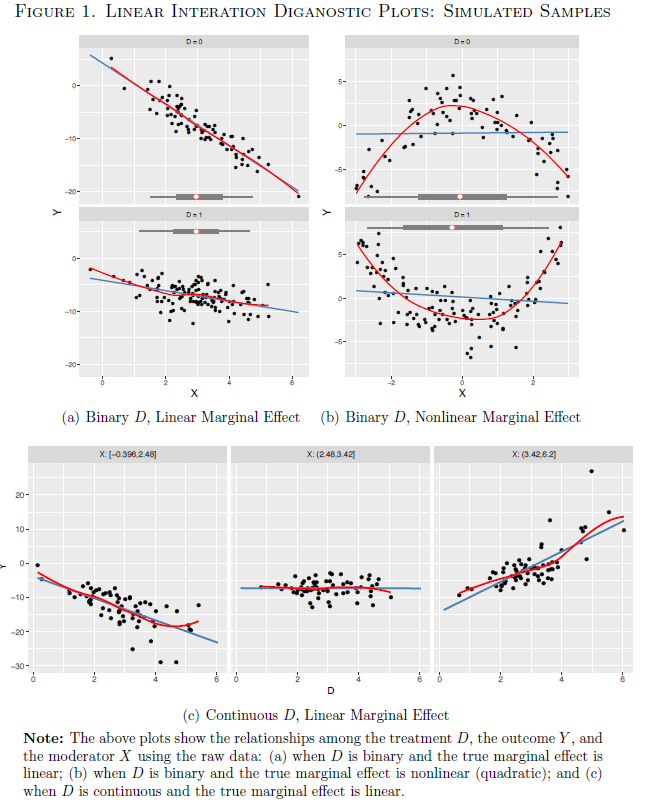LID plots

## 3. 估计量

### 3.1 箱型估计量（Binning Estimator）

1. 如果 （1）线性回归线与 L,M,H 不存在显著差异（可根据 Wald 检验判断） （2）L,M,H 在整个数据区间内分布比较均匀，不是集中在某个区域 说明满足 LIE 假设和共同支持条件，线性模型提供的是一致和有效估计量。

2. 如果箱型估计量 L,M,H 偏离原模型的拟合线，分布在其两侧，说明条件边际作用非线性，拒绝 LIE 假设。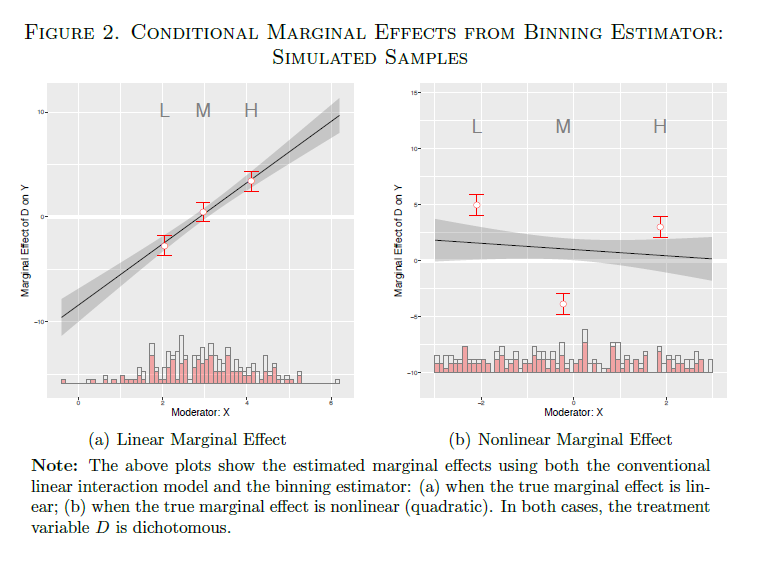Binning Estimator

### 3.2 核估计量（Kernel Estimator）

1. 如果核估计量结果接近一条直线，则满足 LIE 假设；如果弯曲程度很大，那么 LIE 假设不满足，线性模型结果不一致。

2. 置信区间越宽的区域，越缺乏共同支持。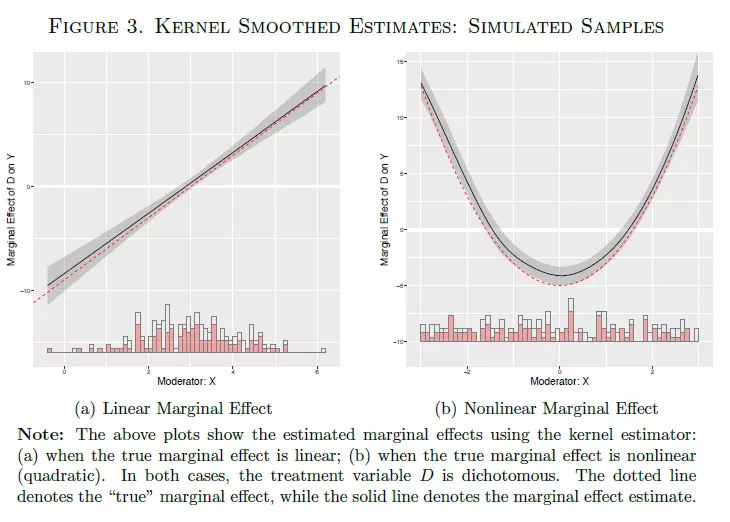Kernel Estimator

## 4. 结论

1. 画 LID 图以检查原始数据，包括 $X|D$ 和 $D|X$ 两个方面。
2. 参考箱型估计量。
3. 参考核估计量。
4. 谨慎使用线性交互模型。得到箱型或核估计量支持才使用线性模型，并且应当在有充分的共同支持的数据区域计算边际效应。建立线性模型后还要再参考 Brambor, Clark and Golder (2006)一文的使用指导。

## 5. interflex 命令介绍

`````` interflex outcome treat moderator [covar] [if] [in] [weight] [, options]
//选项解释
*- fe(varlist)             specify fixed effects variables
*- type(string)            specify the estimation strategy, including binning (default), linear, and kernal
*- vce(vcetype)            specify the variance–covariance estimator; vcetype can be homoscedastic (default), robust, cluster, bootstrap, or off
*-  bw(real)                set the bandwidth for kernel estimations
``````

• `fe(varlist)` 设定固定效应变量；
• `type(string)` 设定估计方法，箱型（默认）、线性和核估计量；
• `vce(vcetype)` 设定协方差矩阵类型，默认是同方差，也可以选择 robust , cluster 等；
• `bw(real)` 可以设定核估计带宽值，以提高效率。

## 6. 应用

### 6.1 线性二分类变量处理效应

``````. use interflex_s1.dta, clear
. twoway (sc Y X) (lowess Y X), by(D) // Plot the raw data
. interflex Y D X Z1 // Estimate a linear interaction model
``````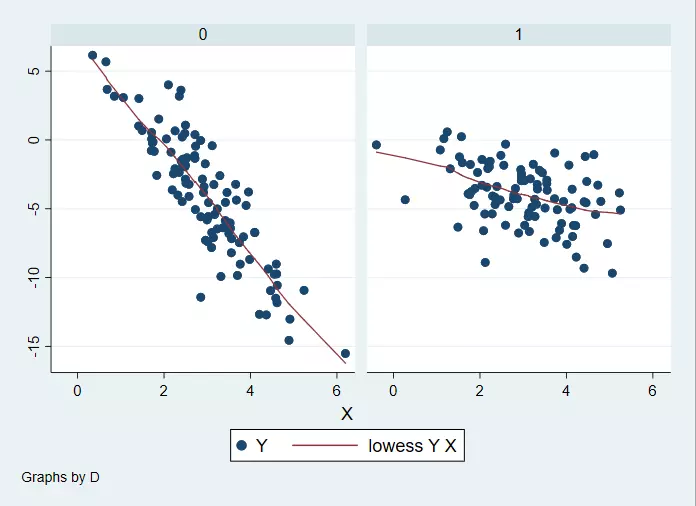scatter1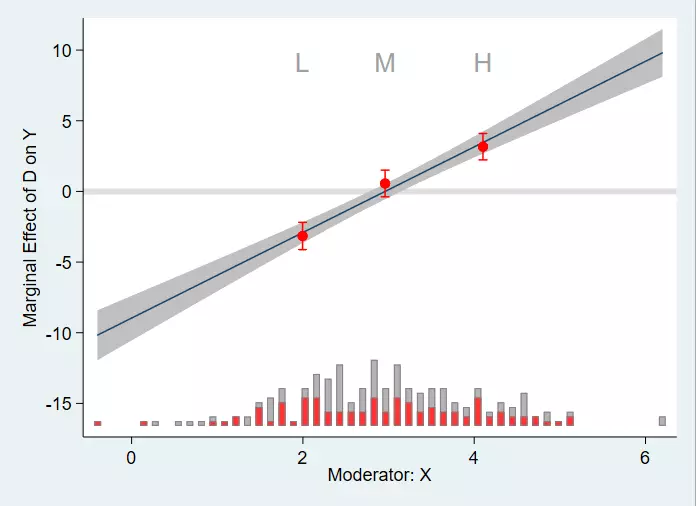线性二分类变量处理效应

### 6.2 线性连续变量处理效应

``````. use interflex_s2.dta, clear
. egen Xbin = cut(X), group(3)       //生成类别变量
. twoway (sc Y D) (lowess Y D), by(Xbin)
. interflex Y D X Z1, type(kernel) bw(5.0)
``````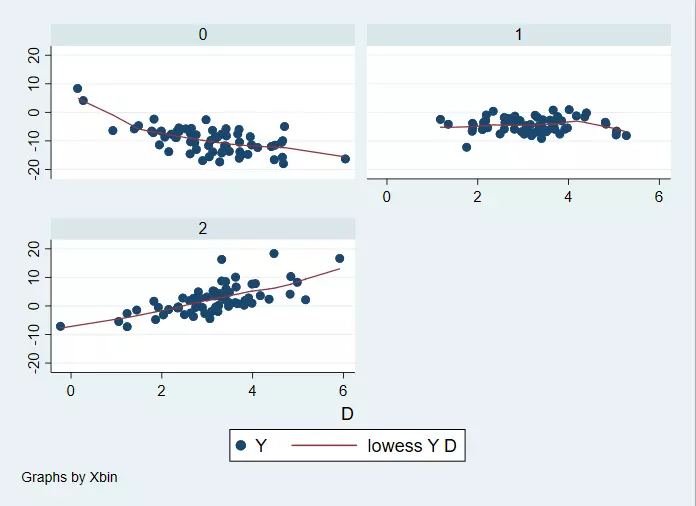scatter2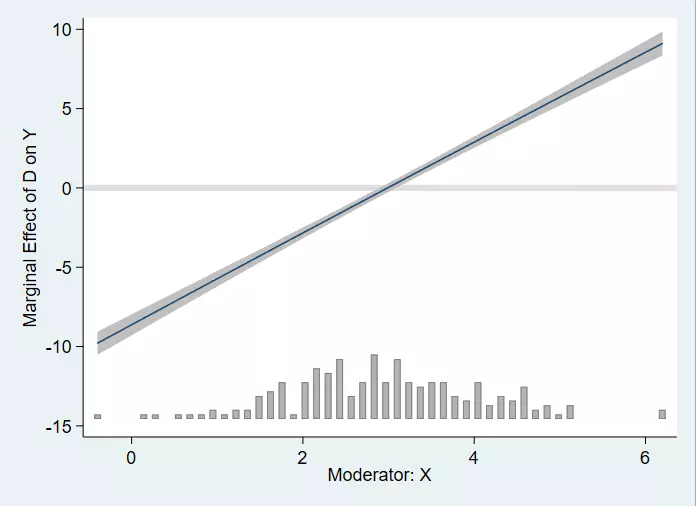线性连续变量处理效应

### 6.3 非线性二分类变量处理效应

``````use interflex_s3.dta, clear
twoway (sc Y X) (lowess Y X), by(D)
``````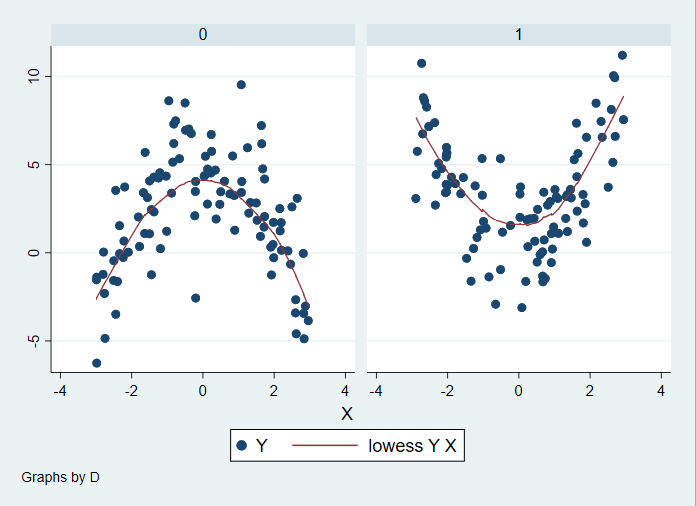sactter3

``````interflex Y D X Z1, vce(r)
p value of Wald test: 0.0000
``````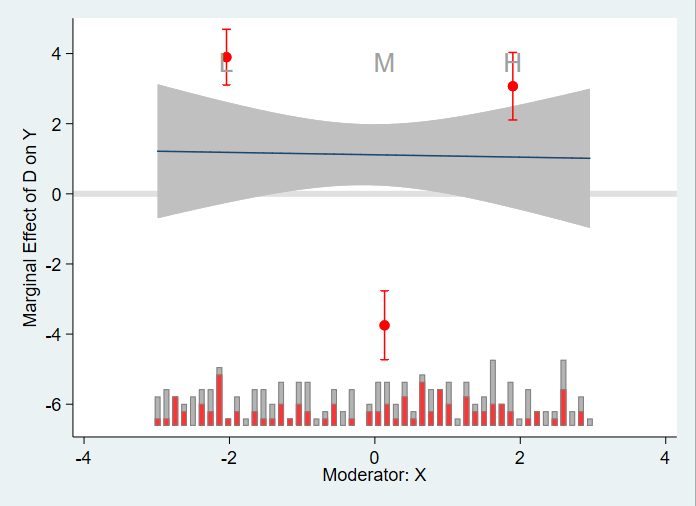Binning1

`````` interflex Y D X Z1, type(kernel) bw(0.345)
``````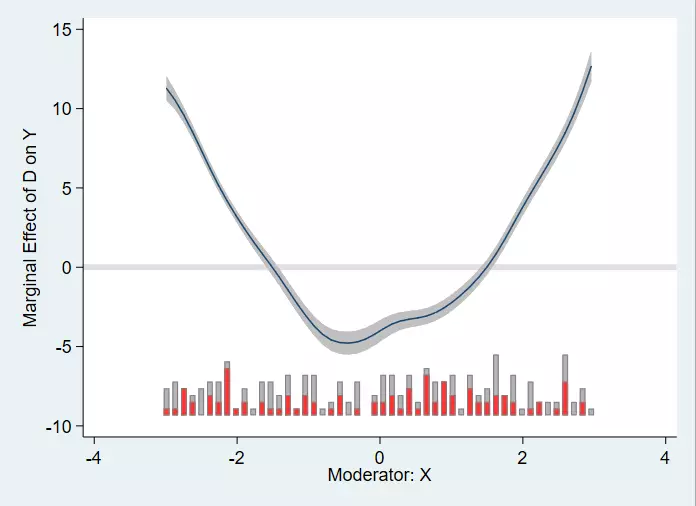kernel1

### 6.4 固定效应对结果的影响

``````use interflex_s4.dta, clear
//控制 组别和年份 固定效应
//画出原始数据
twoway (sc Y X) (lowess Y X), by(D)
interflex Y D X Z1, cl(group)
//如果固定效应没有被控制，那么箱型统计量会有较大的置信区间
// 控制固定效应
interflex Y D X Z1, fe(group year) cl(group)
``````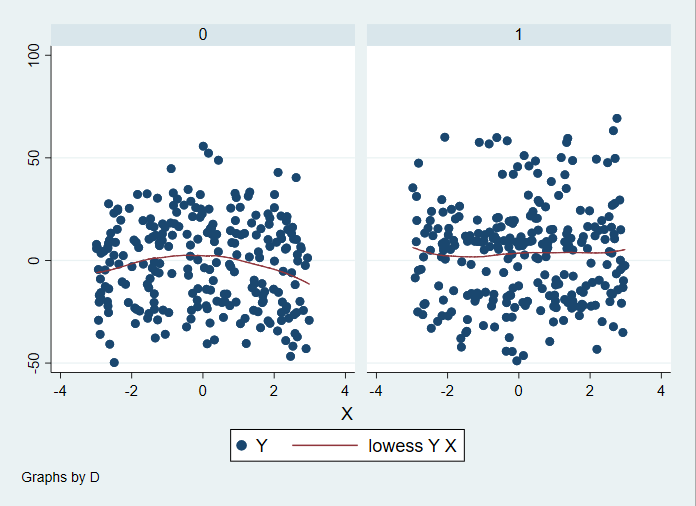scatter4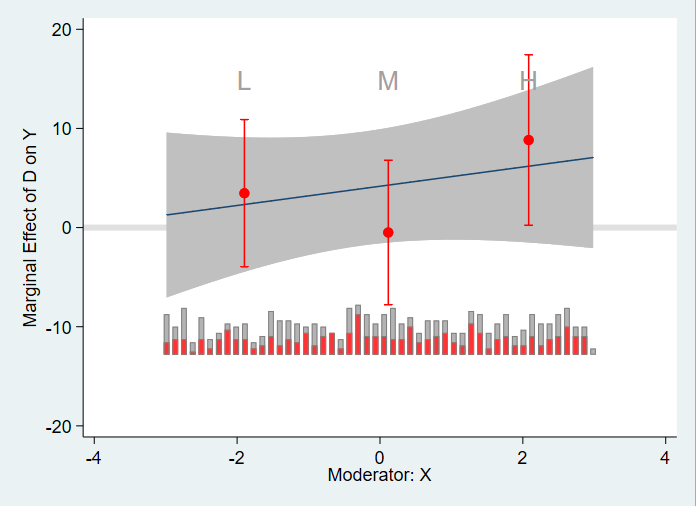未控制固定效应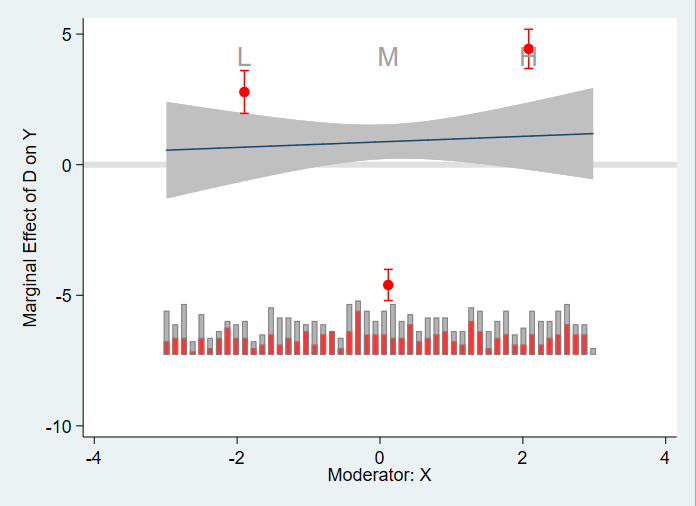控制固定效应

## 相关课程

http://lianxh.duanshu.com

### 课程一览

Stata数据清洗 游万海 直播, 2 小时，已上线

Note: 部分课程的资料，PPT 等可以前往 连享会-直播课 主页查看，下载。

#### 关于我们

• Stata连享会 由中山大学连玉君老师团队创办，定期分享实证分析经验。直播间 有很多视频课程，可以随时观看。
• 连享会-主页知乎专栏，300+ 推文，实证分析不再抓狂。
• 公众号推文分类： 计量专题 | 分类推文 | 资源工具。推文分成 内生性 | 空间计量 | 时序面板 | 结果输出 | 交乘调节 五类，主流方法介绍一目了然：DID, RDD, IV, GMM, FE, Probit 等。
• 公众号关键词搜索/回复 功能已经上线。大家可以在公众号左下角点击键盘图标，输入简要关键词，以便快速呈现历史推文，获取工具软件和数据下载。常见关键词：`课程, 直播, 视频, 客服, 模型设定, 研究设计, stata, plus, 绘图, 编程, 面板, 论文重现, 可视化, RDD, DID, PSM, 合成控制法`连享会主页 lianxh.cn

✏ 连享会学习群-常见问题解答汇总：
https://gitee.com/arlionn/WD